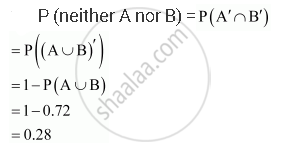Share

# Given Two Independent Events a and B Such that P (A) = 0.3, P (B) = 0.6. Find P (Neither a Nor B) - CBSE (Commerce) Class 12 - Mathematics

#### Question

Given two independent events A and B such that P (A) = 0.3, P (B) = 0.6. Find P (neither A nor B)

#### Solution

It is given that P (A) = 0.3 and P (B) = 0.6

Also, A and B are independent events.Is there an error in this question or solution?

#### Video TutorialsVIEW ALL 

Solution Given Two Independent Events a and B Such that P (A) = 0.3, P (B) = 0.6. Find P (Neither a Nor B) Concept: Independent Events.
S